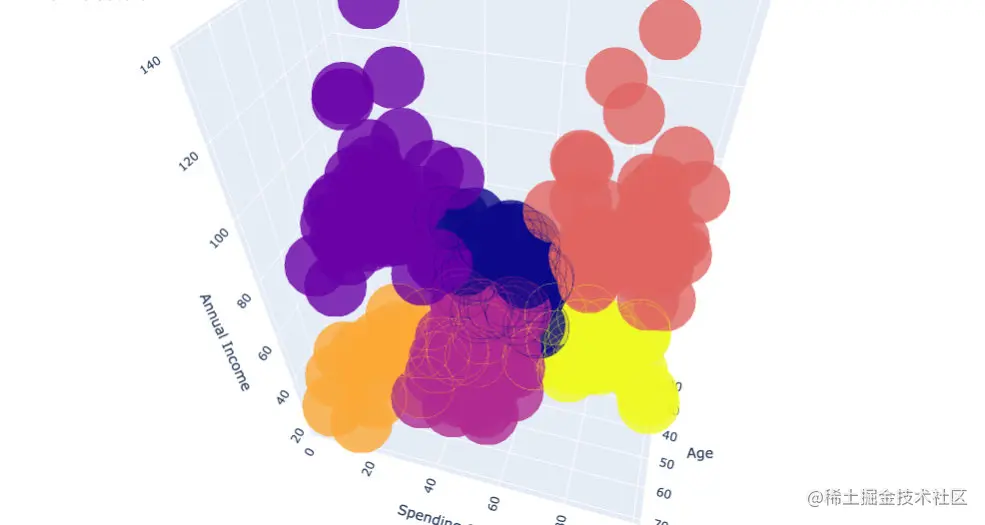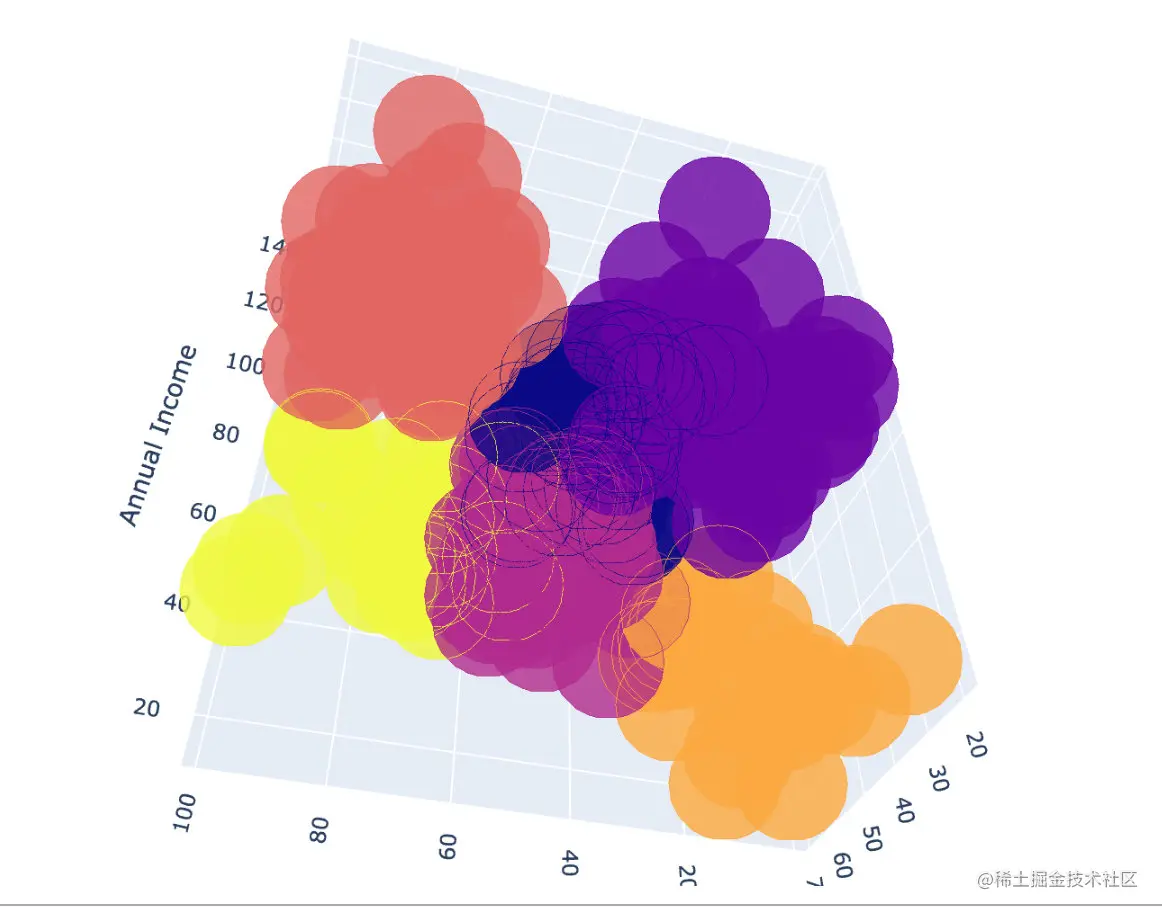# Plotly + pandas + sklearn: shoot the first shot of kaggle

official account ： Youer cottage
author ：Peter
edit ：Peter

Hello everyone , I am a Peter~

Many readers have asked me ： Is there any better data analysis 、 Cases of data mining ？ The answer is, of course , All in Kaggle Come on .

It's just that you have to spend time studying , Even playing games .Peter I have no competition experience , But I often go shopping Kaggle, Learn the problem-solving ideas and methods of the big guys in the game .

A good way to record the big guys , It's to improve yourself ,Peter Decided to open a column ：Kaggle Case sharing .

The case analysis will be updated from time to time later , Ideas come from the big guys on the Internet , In especial Top1 The share of ,Peter Mainly responsible for ： Organize your thoughts 、 Learning technology .

Today I decided to share an article about clustering The case of , It uses ： Supermarket user segmentation data set , Please move to the official website address ： The supermarket

For the convenience of everyone to practice , Official account back office reply The supermarket , You can get this data set ~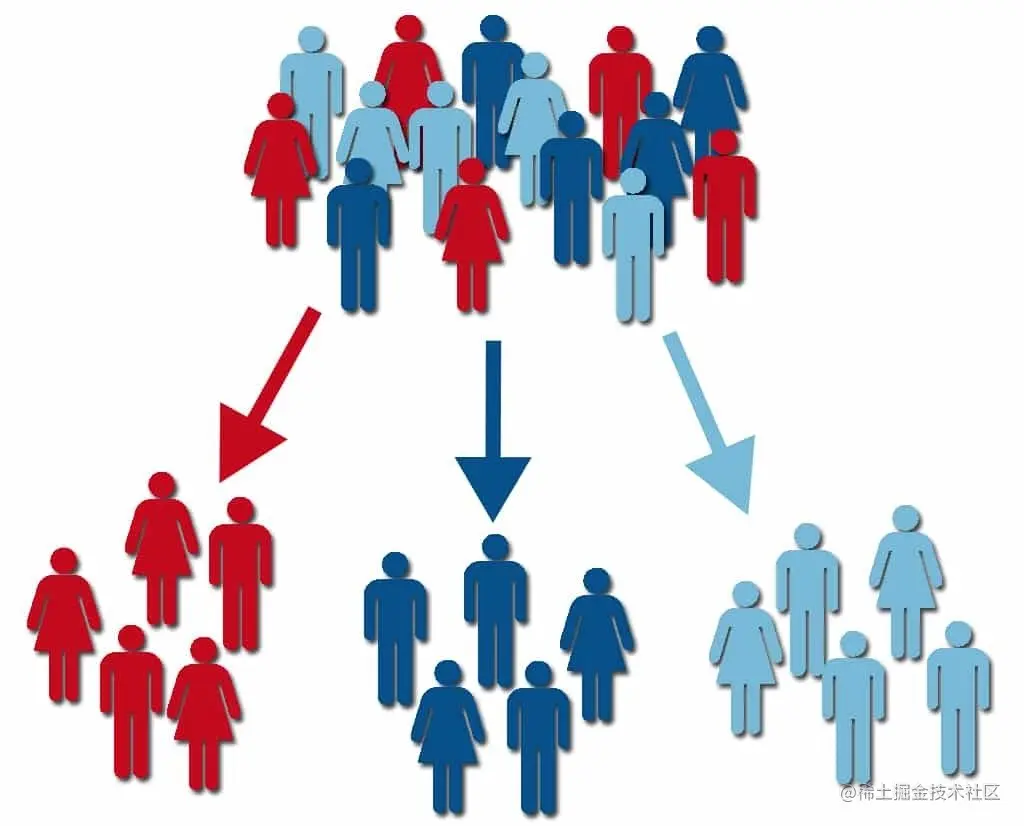Here is the ranking Top1 Of Notebook Source code , Welcome to learn ~

## Import library

``````#  Data processing
import numpy as np
import pandas as pd
# KMeans clustering
from sklearn.cluster import KMeans

#  Drawing library
import matplotlib.pyplot as plt
import seaborn as sns
import plotly as py
import plotly.express as px
import plotly.graph_objects as go
py.offline.init_notebook_mode(connected = True)
Copy code ``````

## data EDA

### Import data

First, let's import the dataset ：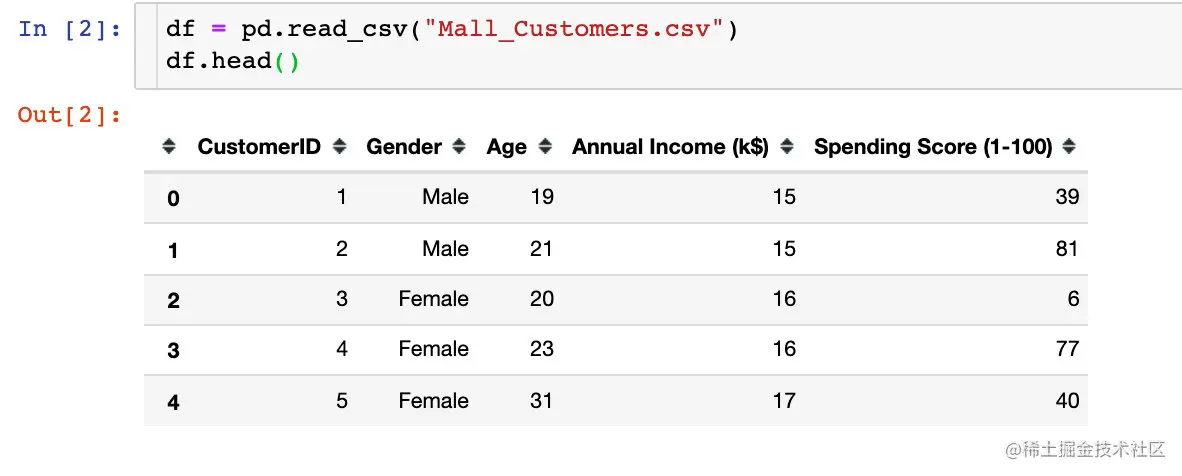We found in the data 5 Attribute fields , It's the customer ID、 Gender 、 Age 、 Average income 、 Consumption level

### Data exploration

1、 Data shape shape

``````df.shape

#  result
(200,5)
Copy code ``````

The total is 200 That's ok ,5 Columns of data

2、 Missing values

``````df.isnull().sum()

#  result
CustomerID                0
Gender                    0
Age                       0
Annual Income (k\$)        0
Spending Score (1-100)    0
dtype: int64
Copy code ``````

You can see ： All fields are complete , No missing value

3、 data type

``````df.dtypes

#  result
CustomerID                 int64
Gender                    object
Age                        int64
Annual Income (k\$)         int64
Spending Score (1-100)     int64
dtype: object
Copy code ``````

In the field type , Except for gender Gender Is string , Others are int64 Numerical type of

4、 Describe statistics

Description statistics are mainly used to view Numerical type The values of the relevant statistical parameters of the data , such as ： Number 、 The median 、 variance 、 The most value 、 Quartile, etc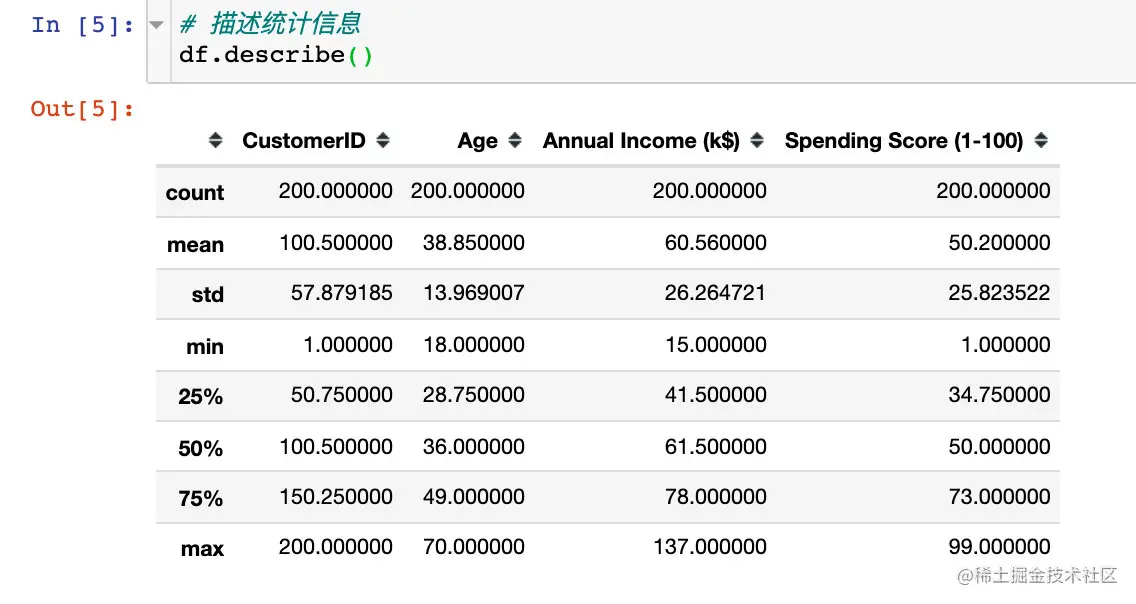For the convenience of subsequent data processing and display , Deal with two points ：

``````# 1、 Set the drawing style
plt.style.use("fivethirtyeight")

# 2、 Take out the key analysis 3 A field
cols = df.columns[2:].tolist()
cols
#  result
['Age', 'Annual Income (k\$)', 'Spending Score (1-100)']
Copy code ``````

## 3 Attribute histogram

see 'Age'、 'Annual Income (k\$)'、 'Spending Score (1-100)' Histogram , Observe the overall distribution ：

``````#  mapping
plt.figure(1,figsize=(15,6))  #  Canvas size
n = 0

for col in cols:
n += 1 #  Subgraph location
plt.subplot(1,3,n)  #  Subgraphs
sns.distplot(df[col],bins=20)  #  Draw histogram
plt.title(f'Distplot of {col}')  #  title
plt.show()  #  The graphics
Copy code ``````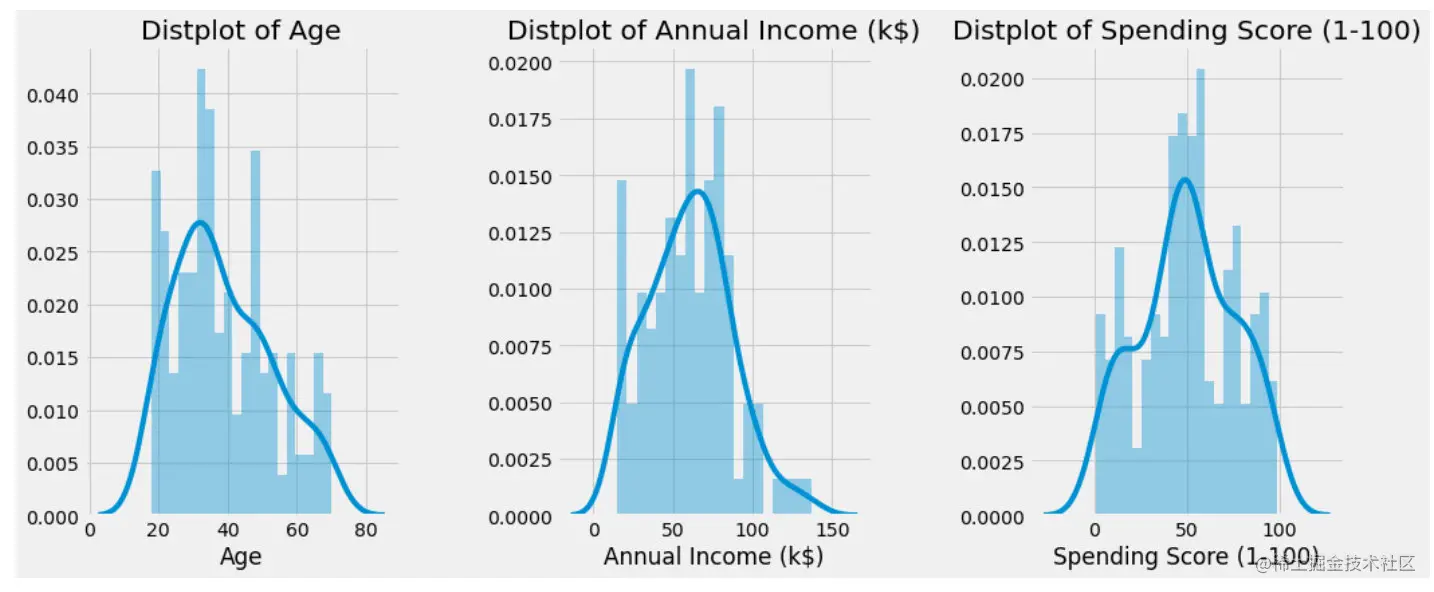## The gender factor

### Gender statistics

See how many men and women in this data set . In the follow-up, we will consider whether gender has an impact on the overall analysis .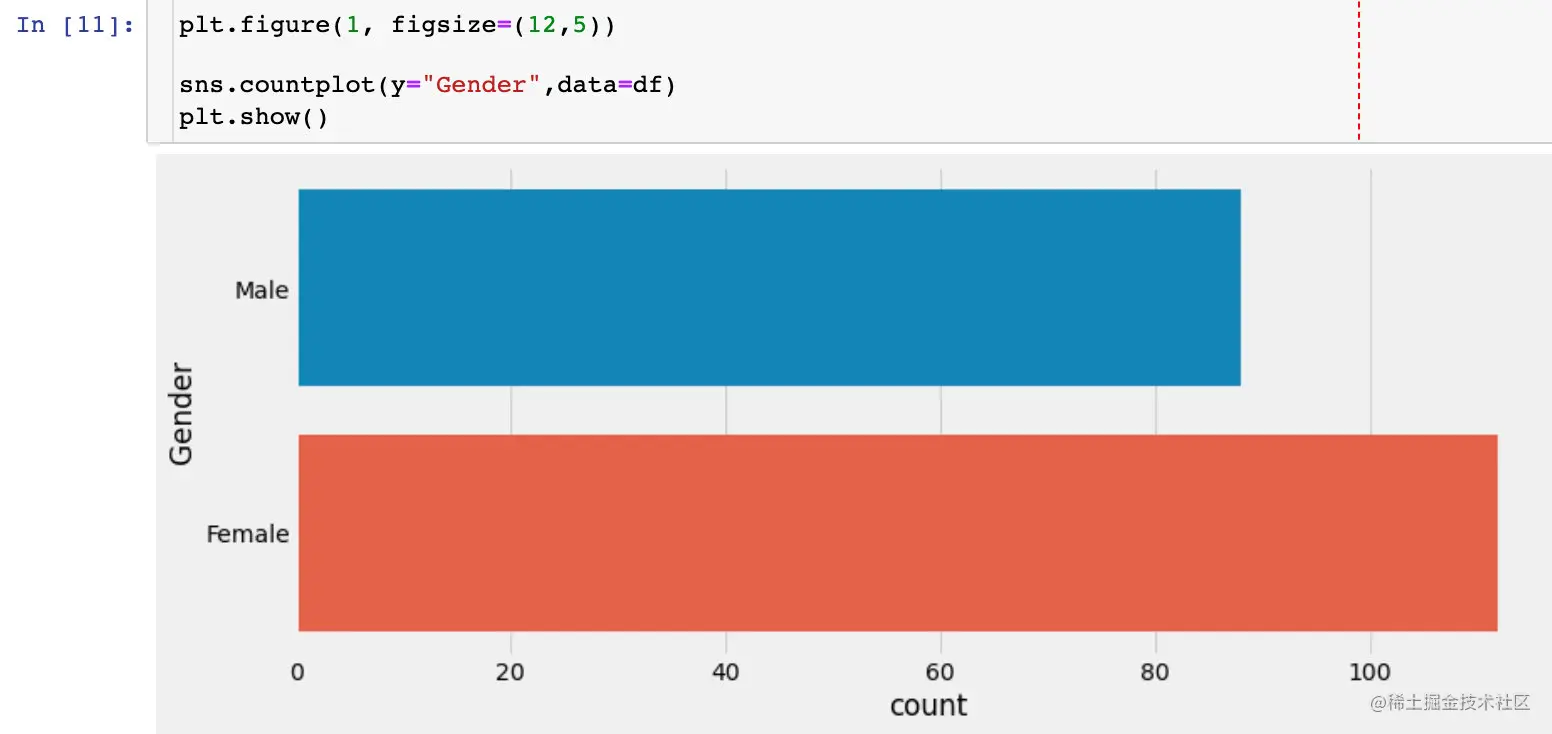### Data distribution under different genders

``````sns.pairplot(df.drop(["CustomerID"],axis=1),
hue="Gender",  #  Grouping field
aspect=1.5)
plt.show()
Copy code ``````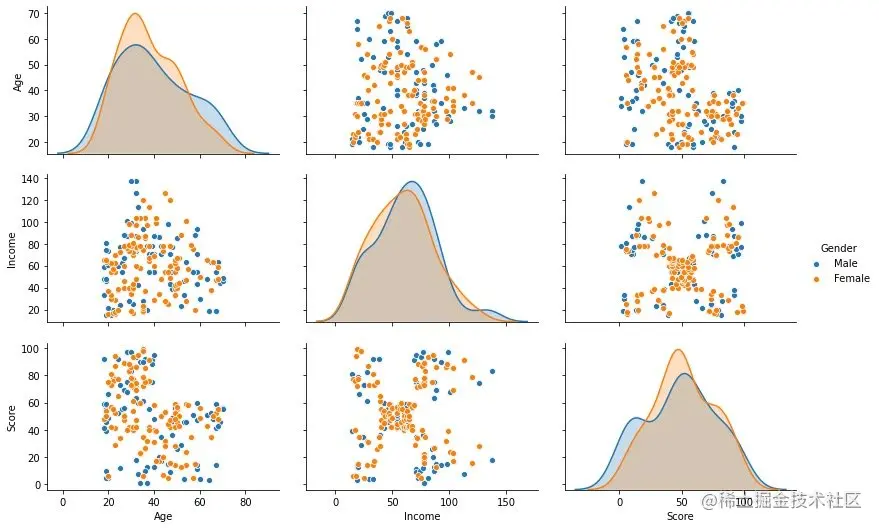Through the bivariate distribution diagram above , We observed that ： Gender factors affect other 3 Fields have little effect

### The relationship between age and average income under different gender

``````plt.figure(1,figsize=(15,6))  #  Drawing size

for gender in ["Male", "Female"]:
plt.scatter(x="Age", y="Annual Income (k\$)", #  Specify two fields for analysis
data=df[df["Gender"] == gender],  #  Data to be analyzed , Some gender Next
s=200,alpha=0.5,label=gender  #  The size of the scatter 、 transparency 、 Label classification
)

#  Horizontal and vertical axis 、 Title Setting
plt.xlabel("Age")
plt.ylabel("Annual Income (k\$)")
plt.title("Age vs Annual Income w.r.t Gender")
#  The graphics
plt.show()
Copy code ``````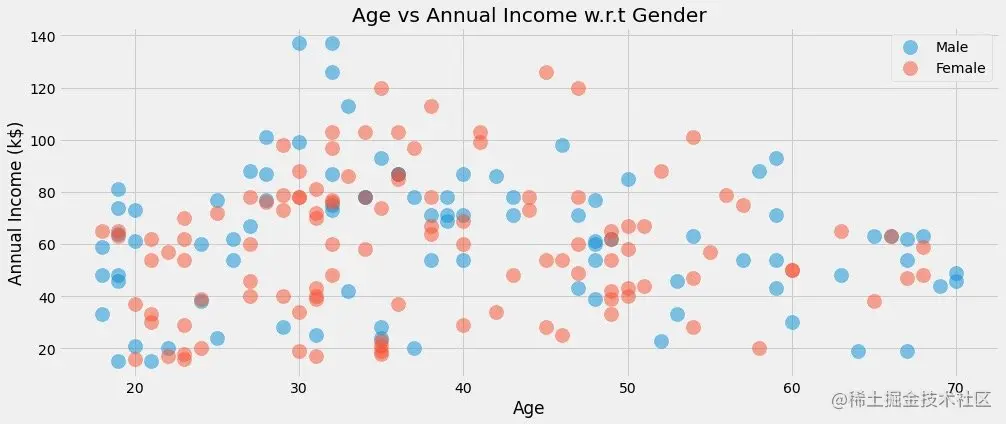### The relationship between average income and consumption score under different gender

``````plt.figure(1,figsize=(15,6))

for gender in ["Male", "Female"]:  #  Please refer to the above for explanation
plt.scatter(x = 'Annual Income (k\$)',y = 'Spending Score (1-100)',
data=df[df["Gender"] == gender],
s=200,alpha=0.5,label=gender)

plt.xlabel('Annual Income (k\$)')
plt.ylabel('Spending Score (1-100)')
plt.title("Annual Income vs Spending Score w.r.t Gender")
plt.show()
Copy code ``````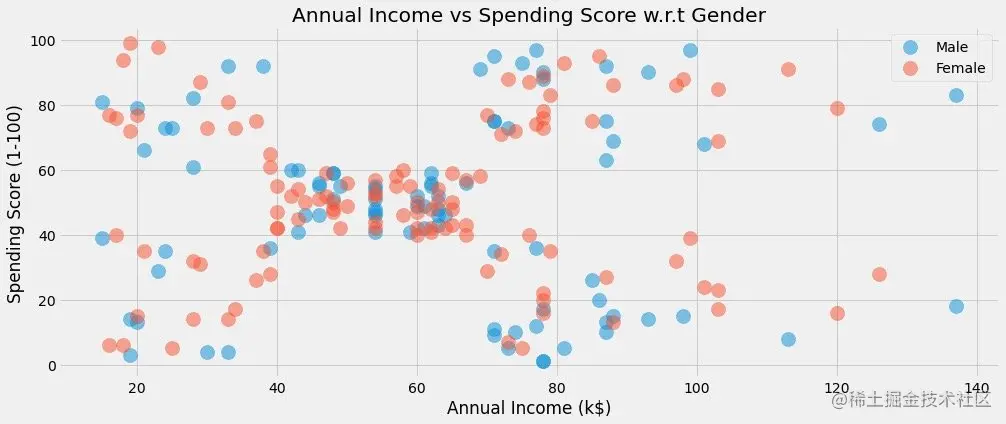### Data distribution under different genders

Observe the data distribution through violin diagram and cluster scatter diagram ：

``````#  Clustering scatter graph ：Swarmplots
#  Violin chart ：violinplot

plt.figure(1,figsize=(15,7))
n = 0

for col in cols:
n += 1  #  Subgraph order
plt.subplot(1,3,n)  #  The first n Subtext
#  Draw a col The following two graphics , adopt Gender Display in groups
sns.violinplot(x=col,y="Gender",data=df,palette = "vlag")
sns.swarmplot(x=col, y="Gender",data=df)
#  Axis and title settings
plt.ylabel("Gender" if n == 1 else '')
plt.title("Violinplots & Swarmplots" if n == 2 else '')

plt.show()
Copy code ``````

give the result as follows ：

• See different Gender The distribution of different fields
• See if there are outliers 、 Outliers, etc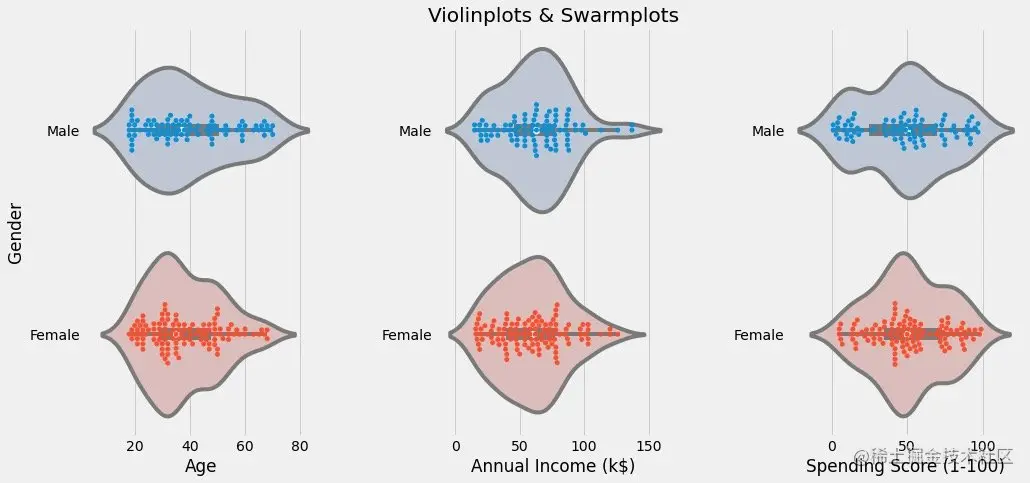## Attribute correlation analysis

It is mainly to observe the regression between two attributes ：

``````cols = ['Age', 'Annual Income (k\$)', 'Spending Score (1-100)']  #  this 3 Correlation analysis of attributes
Copy code ``````
``````plt.figure(1,figsize=(15,6))
n = 0

for x in cols:
for y in cols:
n += 1  #  Every cycle n increase , The subgraph moves once
plt.subplot(3,3,n)  # 3*3 Matrix , The first n A graph
plt.subplots_adjust(hspace=0.5, wspace=0.5)  #  Width between subgraphs 、 High parameter
sns.regplot(x=x,y=y,data=df,color="#AE213D")  #  Drawing data and color
plt.ylabel(y.split() + " " + y.split() if len(y.split()) > 1 else y)

plt.show()
Copy code ``````

The specific figure is ：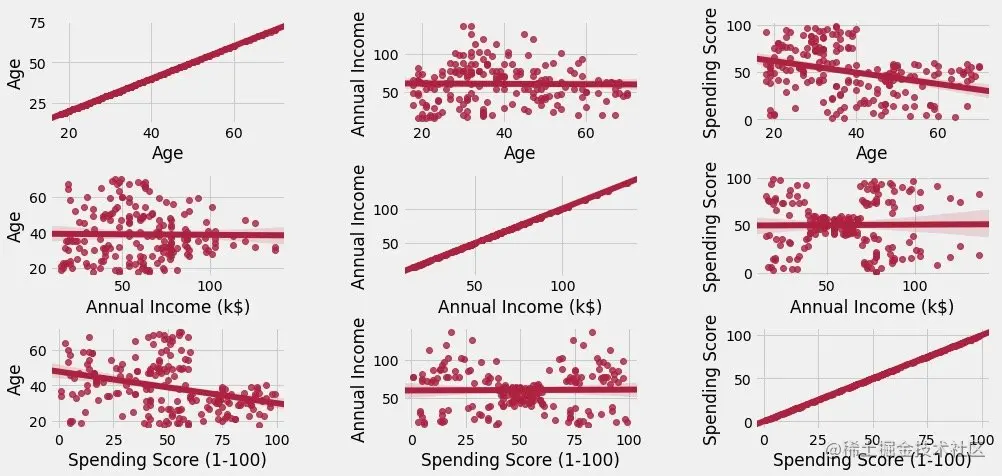The figure above shows two points ：

• The main diagonal is the relationship between itself and itself , In proportion
• Other graphs are between attributes , Scatter distribution with data , At the same time, there are relevant trend charts of simulation

## Clustering between two attributes

The principle and process of clustering algorithm are not explained in detail here , The default is based on

### K It's worth choosing

We plot the data by ELBOW Figure to determine k value . Information broadcast ：

1、 Parameter interpretation from the official website ：scikit-learn.org/stable/modu…

2、 Chinese explanation and reference ：blog.csdn.net/qq_34104548…

``````df1 = df[['Age' , 'Spending Score (1-100)']].iloc[:,:].values  #  Data to be fitted
inertia = []   #  An empty list , Used to store the sum of distances to the center of mass

for k in range(1,11):  # k The default value is 1-10 Between , The empirical value is 5 perhaps 10
algorithm = (KMeans(n_clusters=k,  # k value
init="k-means++",  #  Initial algorithm selection
n_init=10,  #  Number of random runs
max_iter=300,  #  At most iterations
tol=0.0001,  #  Tolerance of minimum error
random_state=111,  #  Random seeds
algorithm="full"))  #  Algorithm to choose  auto、full、elkan
algorithm.fit(df1)  #  Fitting data
inertia.append(algorithm.inertia_)  #  Sum of center of mass
Copy code ``````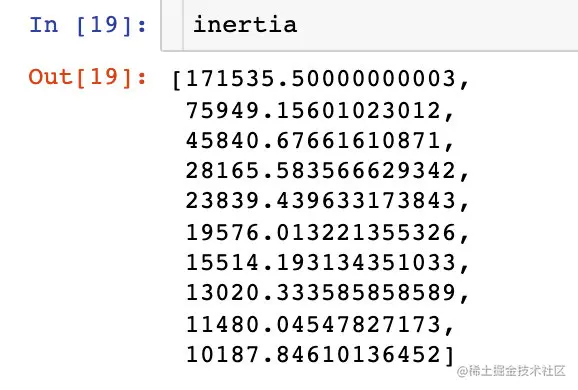Draw out K The relationship between the change of value and the sum of centroid distance ：

``````plt.figure(1,figsize=(15,6))
plt.plot(np.arange(1,11), inertia, 'o')  #  The data is drawn twice , Different marks
plt.plot(np.arange(1,11), inertia, '-', alpha=0.5)

plt.xlabel("Choose of K")
plt.ylabel("Interia")
plt.show()
Copy code ``````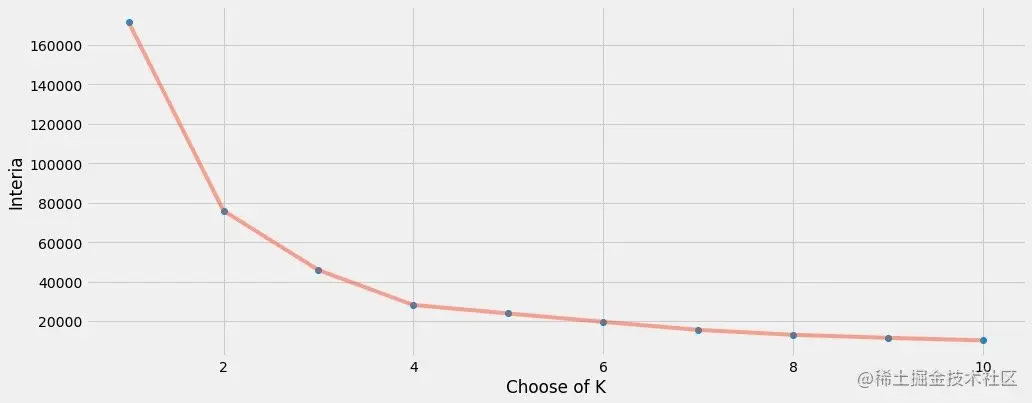In the end, we found out ：k=4 It's more appropriate . So we use k=4 To carry out the real fitting process of data

### Clustering modeling

``````algorithm = (KMeans(n_clusters=4,  # k=4
init="k-means++",
n_init=10,
max_iter=300,
tol=0.0001,
random_state=111,
algorithm="elkan"))
algorithm.fit(df1)  #  Analog data
Copy code ``````

The data are fit After the operation , We got the label label and 4 A center of mass ：

``````labels1 = algorithm.labels_  #  The result of the classification （4 class ）
centroids1 = algorithm.cluster_centers_  #  The position of the final center of mass

print("labels1:", labels1)
print("centroids1:", centroids1)
Copy code ``````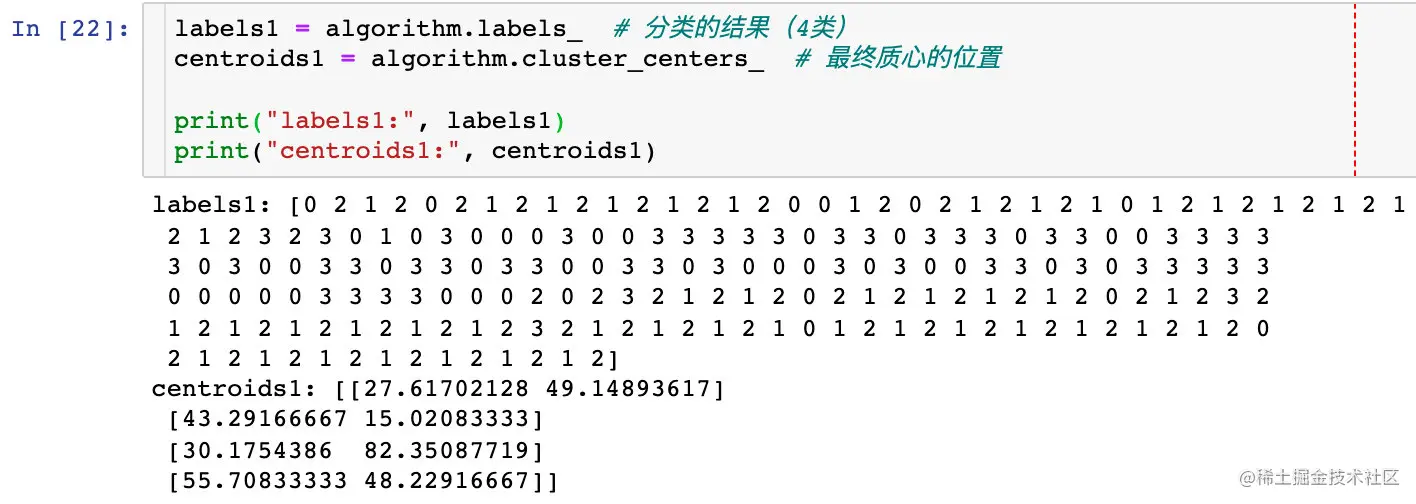In order to show the classification effect of the original data , The case of the official website is the following operation , Personally, I think it's a little cumbersome ：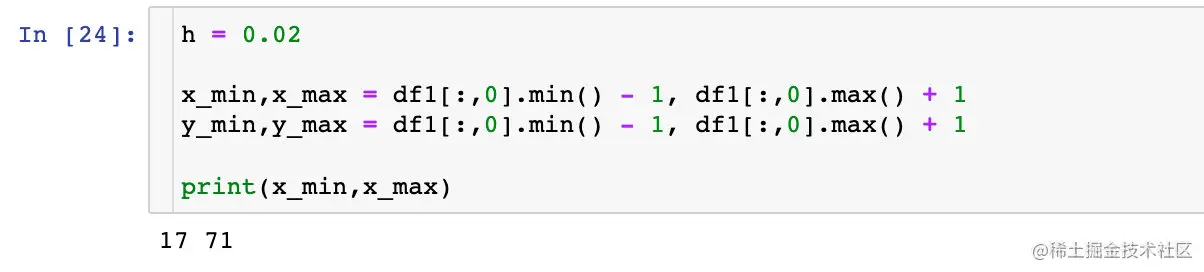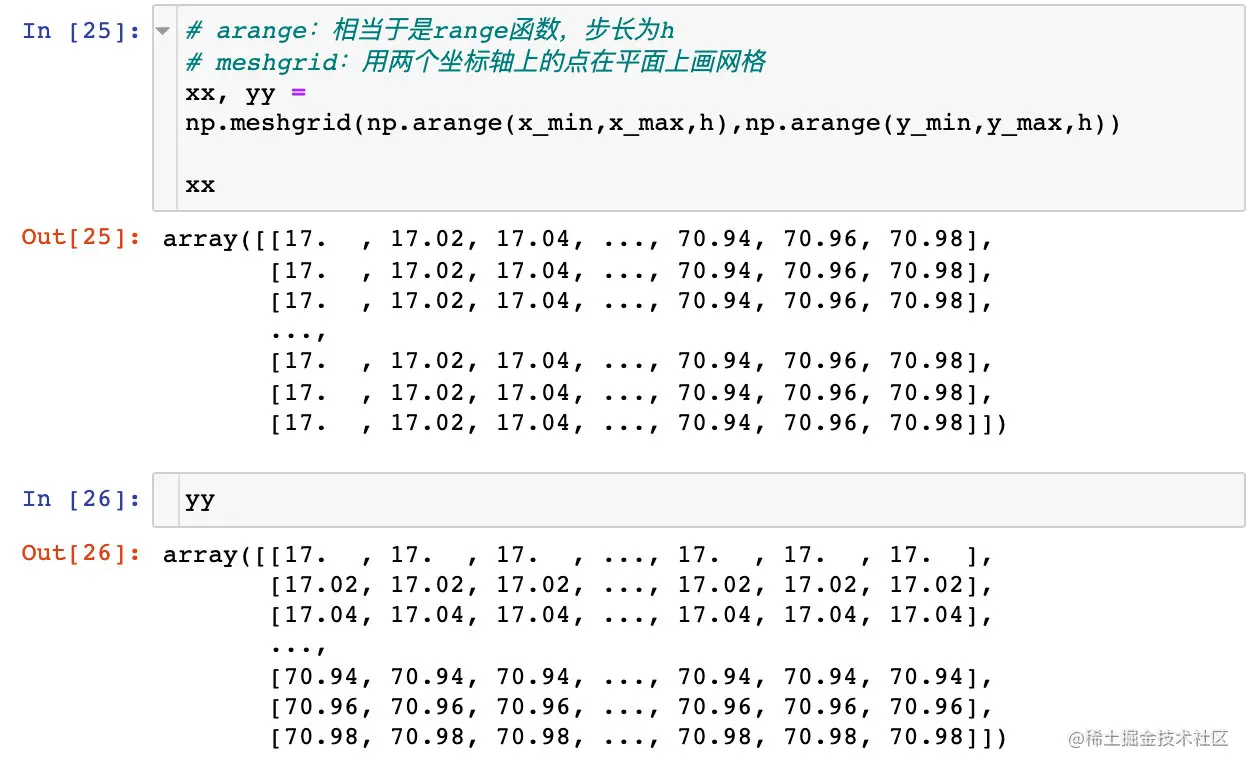Data consolidation ：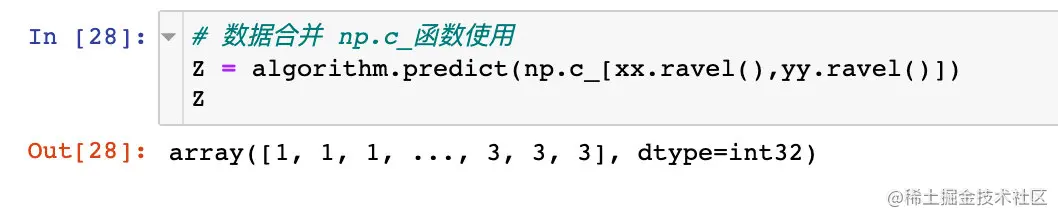Show the classification effect ：

``````plt.figure(1,figsize=(14,5))
plt.clf()

Z = Z.reshape(xx.shape)

plt.imshow(Z,interpolation="nearest",
extent=(xx.min(),xx.max(),yy.min(),yy.max()),
cmap = plt.cm.Pastel2,
aspect = 'auto',
origin='lower')

plt.scatter(x="Age",
y='Spending Score (1-100)',
data = df ,
c = labels1 ,
s = 200)

plt.scatter(x = centroids1[:,0],
y =  centroids1[:,1],
s = 300 ,
c = 'red',
alpha = 0.5)

plt.xlabel("Age")
plt.ylabel("Spending Score(1-100)")

plt.show()
Copy code ``````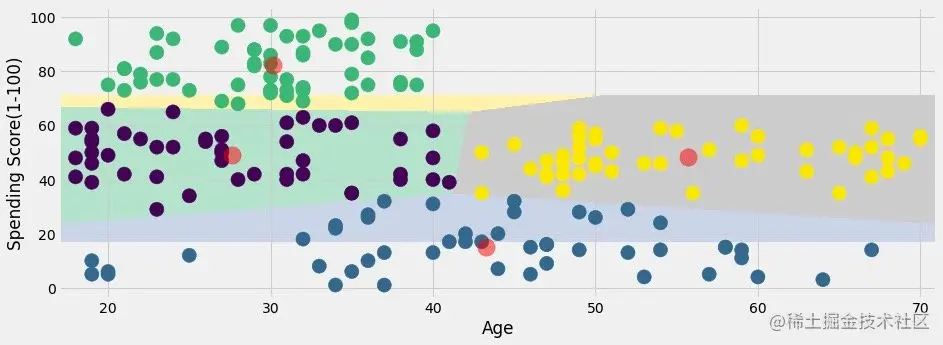If I were , How do you do it? ？ Use, of course Pandas+Plolty To solve the problem perfectly ：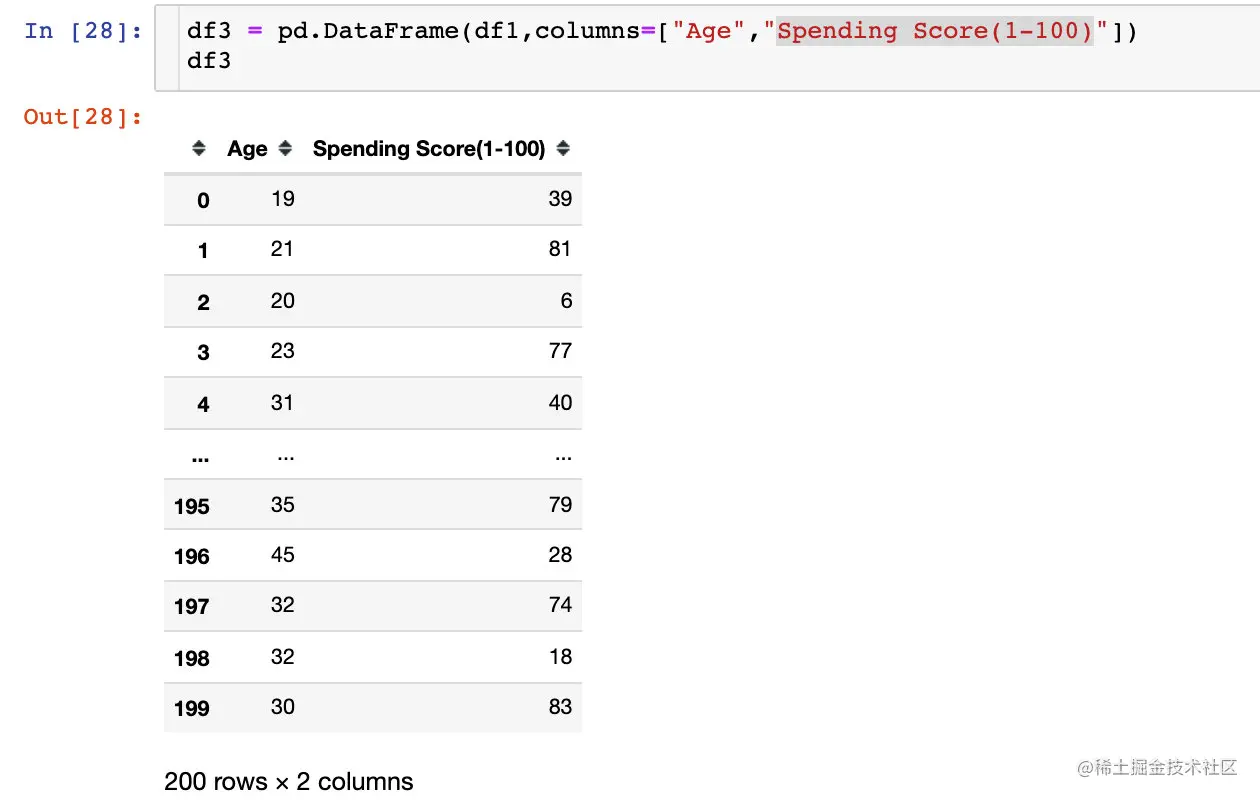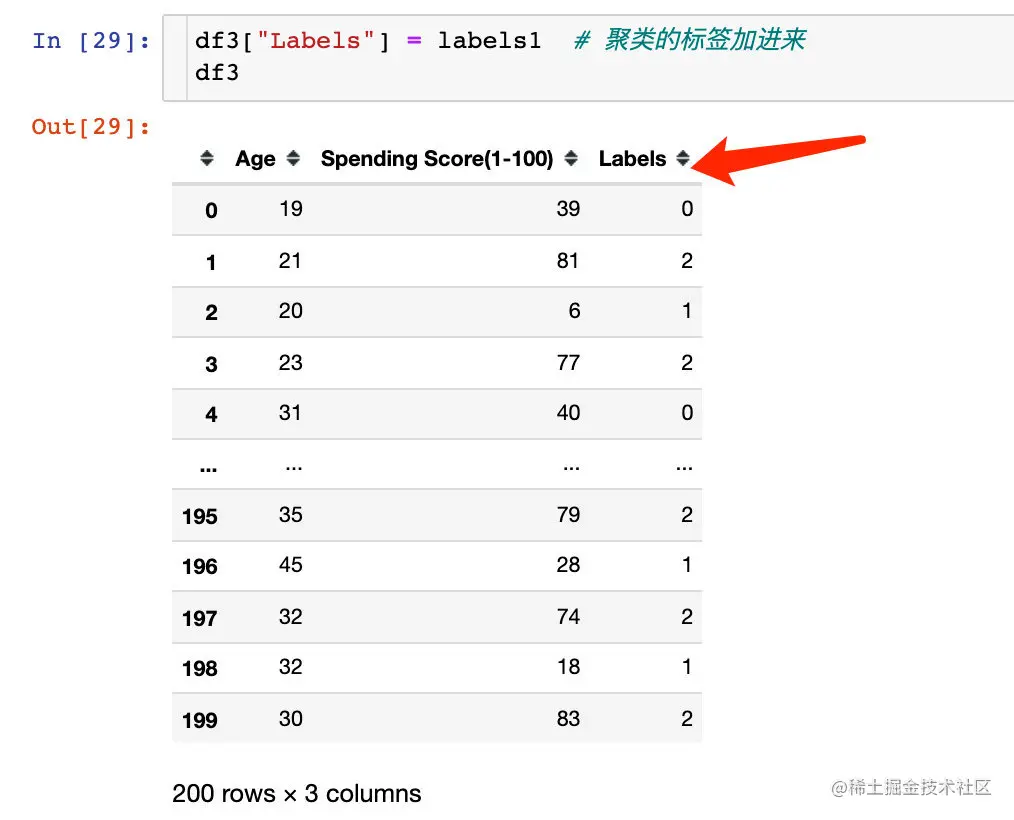Look at the results of classification Visualization ：

``````px.scatter(df3,x="Age",y="Spending Score(1-100)",color="Labels",color_continuous_scale="rainbow")
Copy code ``````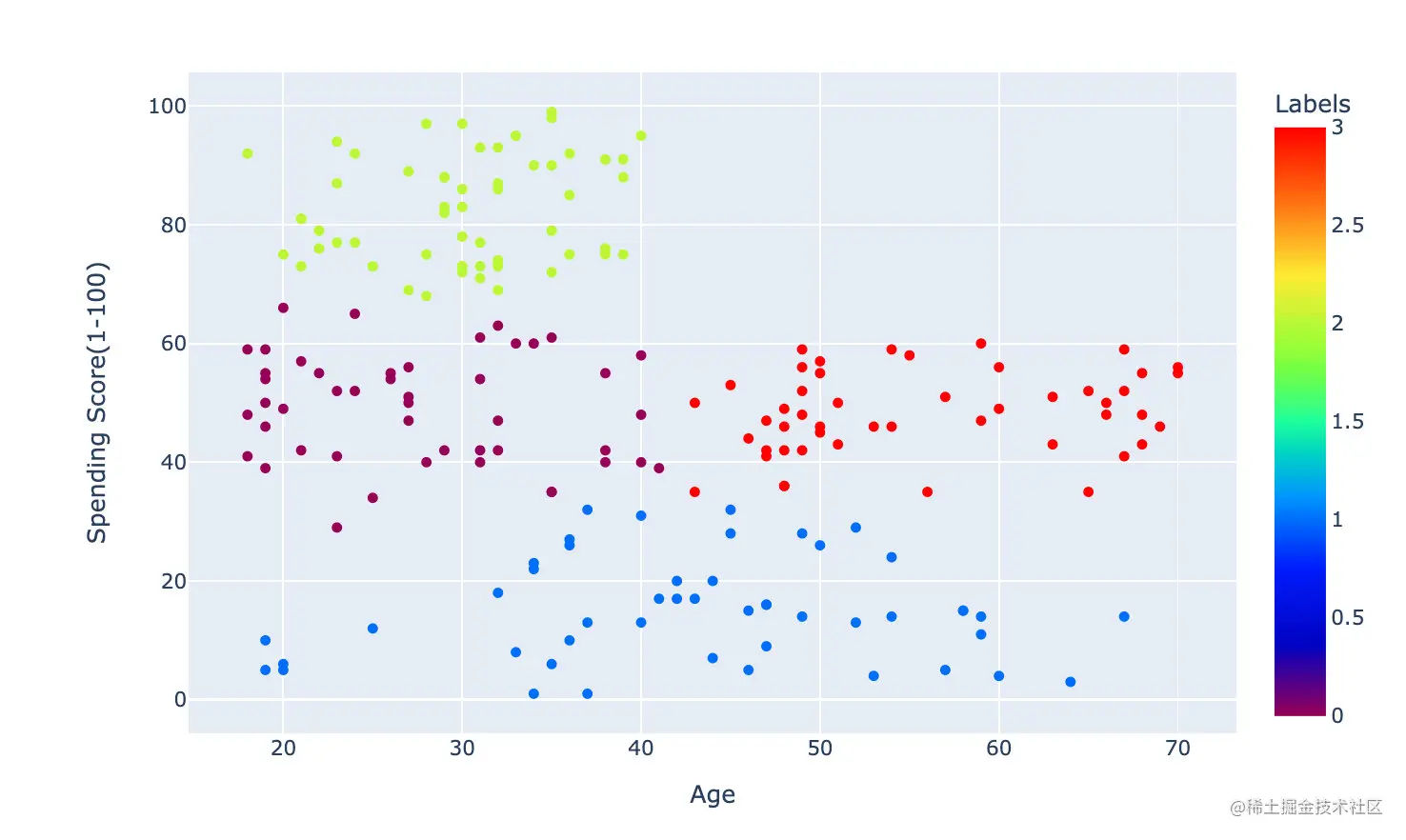The above process is based on Age and Spending Score(1-100) To cluster . Based on the same method on the official website ：Annual Income (k\$) and Spending Score (1-100) Clustering of fields .

The effect is as follows , Divided into 5 Classes ：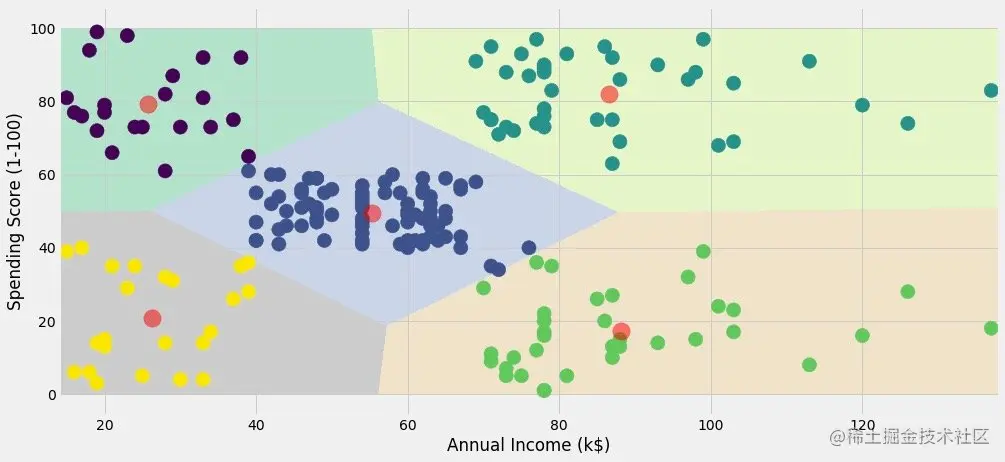## 3 Clustering of attributes

according to Age 、 Annual Income 、 Spending Score To cluster , Final drawing 3 Dimension graphics .

### K It's worth choosing

The methods are the same , Just chose 3 A field （ There's something on it 2 individual ）

``````X3 = df[['Age' , 'Annual Income (k\$)' ,'Spending Score (1-100)']].iloc[: , :].values  #  selection 3 Data of two fields
inertia = []
for n in range(1 , 11):
algorithm = (KMeans(n_clusters = n,
init='k-means++',
n_init = 10 ,
max_iter=300,
tol=0.0001,
random_state= 111  ,
algorithm='elkan') )
algorithm.fit(X3)   #  Fitting data
inertia.append(algorithm.inertia_)
Copy code ``````

Draw an elbow to determine k：

``````plt.figure(1 , figsize = (15 ,6))
plt.plot(np.arange(1 , 11) , inertia , 'o')
plt.plot(np.arange(1 , 11) , inertia , '-' , alpha = 0.5)
plt.xlabel('Number of Clusters') , plt.ylabel('Inertia')
plt.show()
Copy code ``````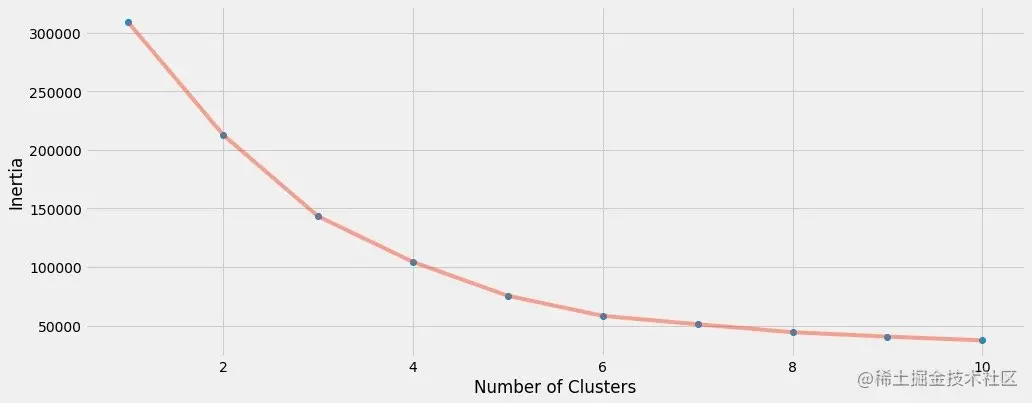We finally chose k=6 To cluster

### Modeling and fitting

``````algorithm = (KMeans(n_clusters=6,  #  affirmatory k value
init="k-means++",
n_init=10,
max_iter=300,
tol=0.0001,
random_state=111,
algorithm="elkan"))
algorithm.fit(df2)

labels2 = algorithm.labels_
centroids2 = algorithm.cluster_centers_

print(labels2)
print(centroids2)
Copy code ``````

Get the label and center of mass ：

``````labels2 = algorithm.labels_
centroids2 = algorithm.cluster_centers_
Copy code ``````

### mapping

3 For dimensional clustering, we finally choose plotly To show ：

``````df["labels2"] = labels2

trace = go.Scatter3d(
x=df["Age"],
y= df['Spending Score (1-100)'],
z= df['Annual Income (k\$)'],
mode='markers',

marker = dict(
color=df["labels2"],
size=20,
line=dict(color=df["labels2"],width=12),
opacity=0.8
)
)

data = [trace]
layout = go.Layout(
margin=dict(l=0,r=0,b=0,t=0),
title="six Clusters",
scene=dict(
xaxis=dict(title="Age"),
yaxis = dict(title  = 'Spending Score'),
zaxis = dict(title  = 'Annual Income')
)
)

fig = go.Figure(data=data,layout=layout)

fig.show()
Copy code ``````

The following is the final clustering effect ：